# Heat transfer By Ankita Yagnik

30. Apr 2018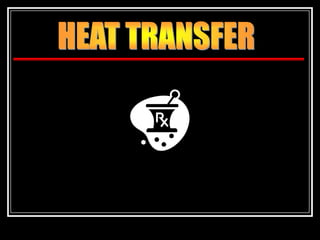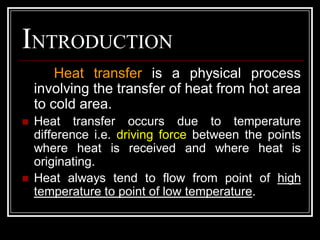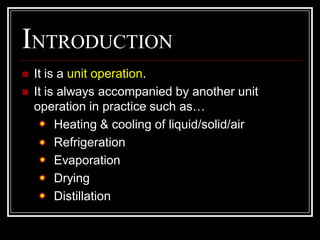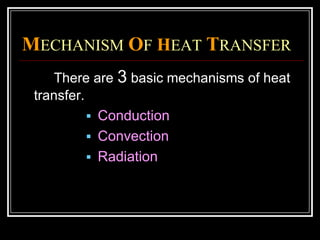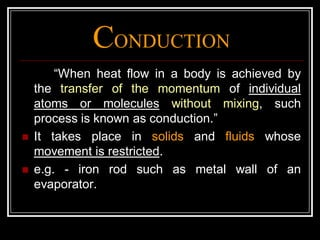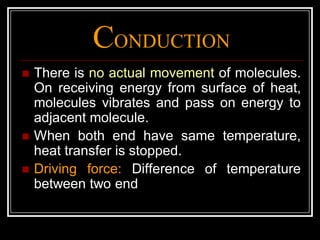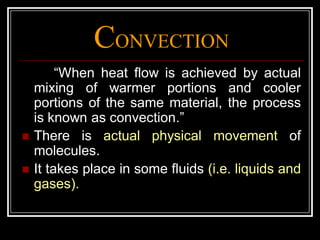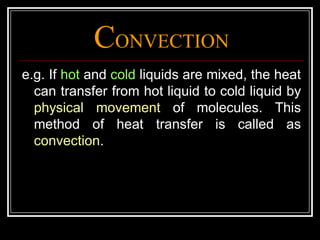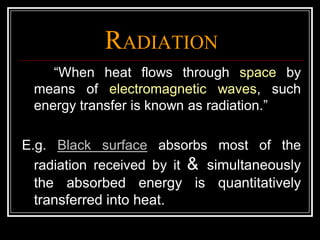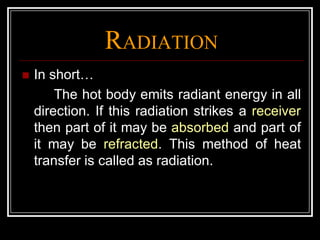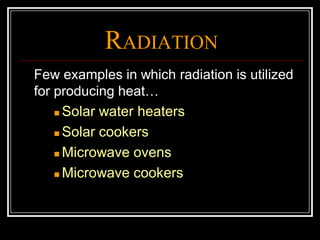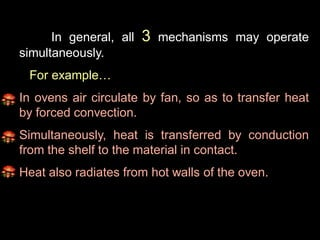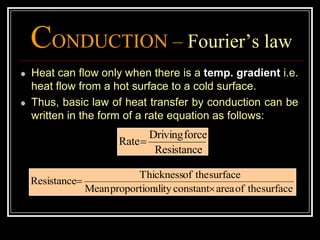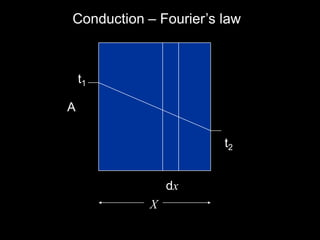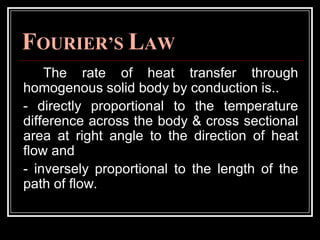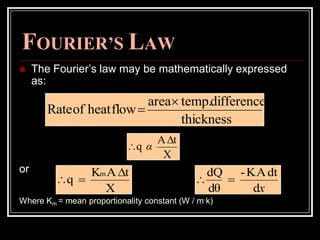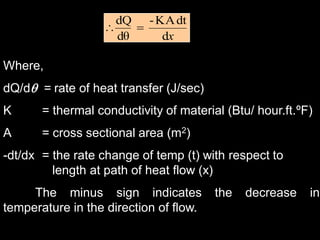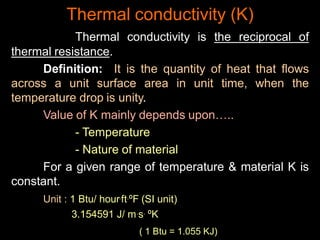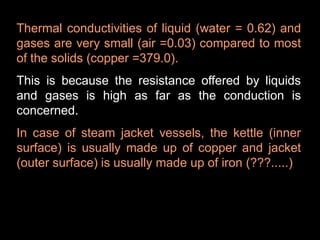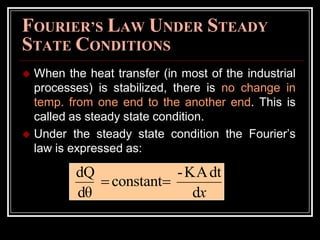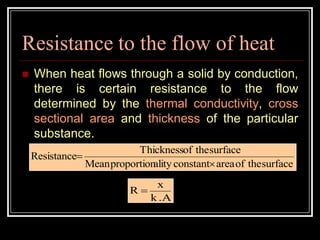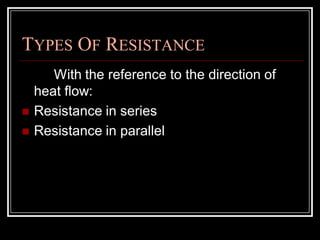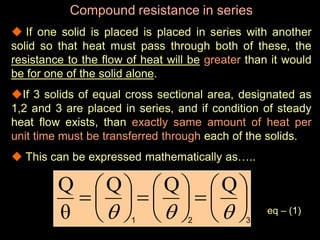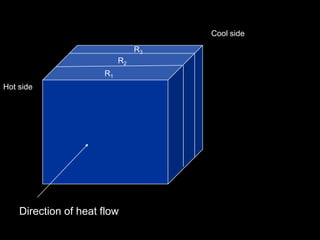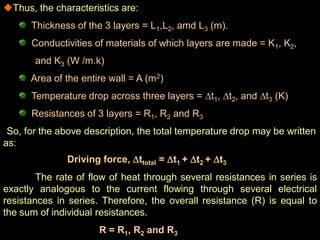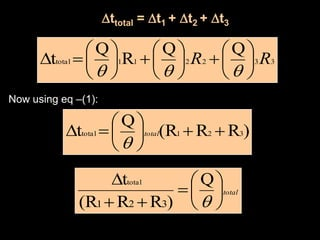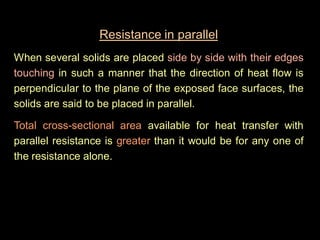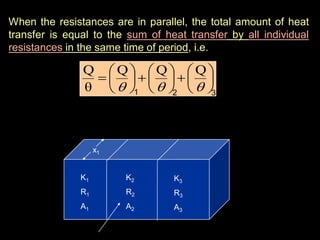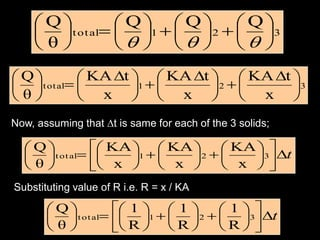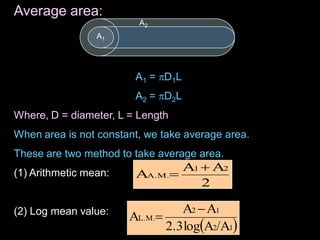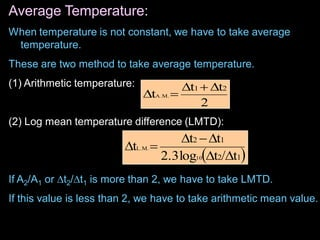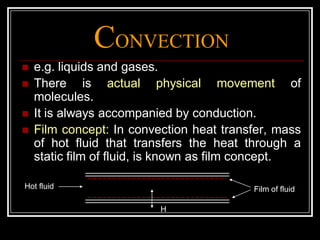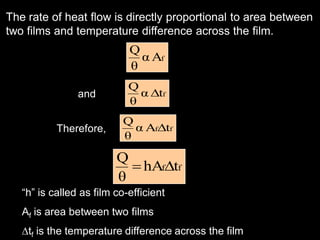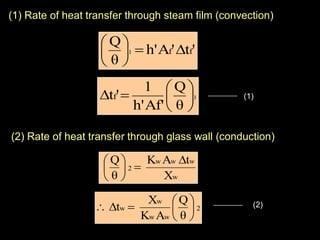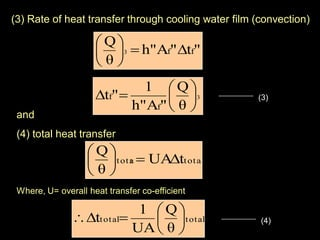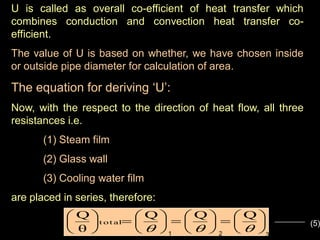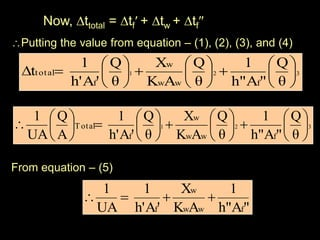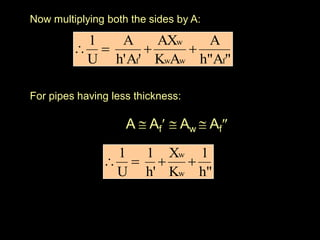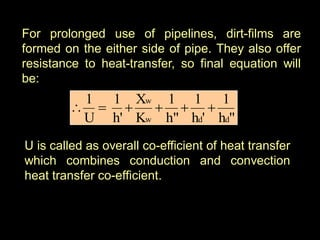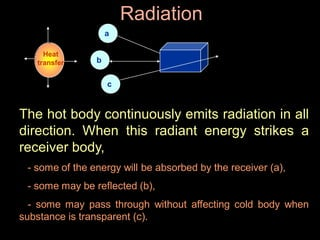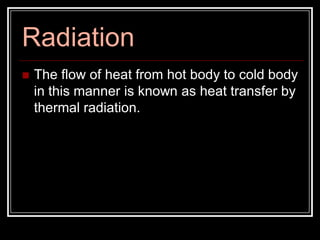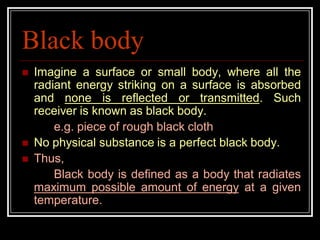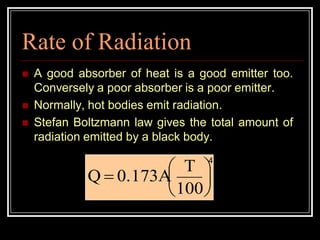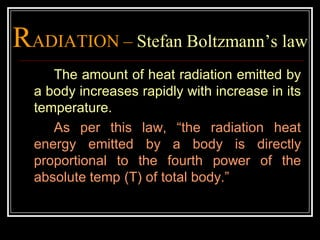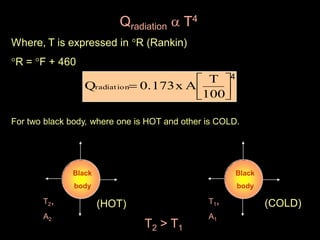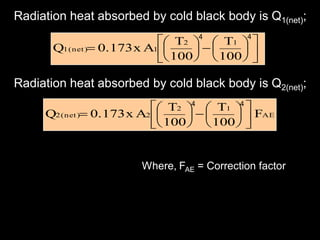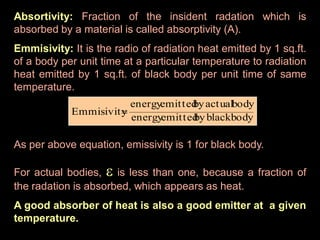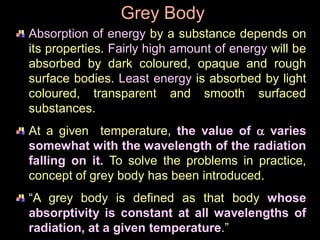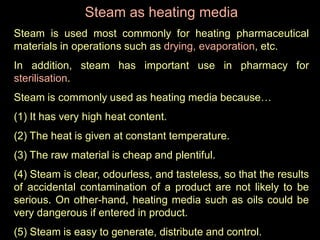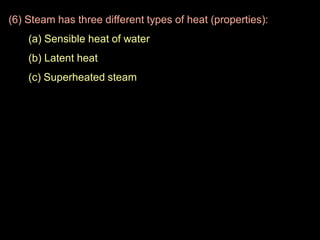1 von 50

### Heat transfer By Ankita Yagnik

• 2. INTRODUCTION Heat transfer is a physical process involving the transfer of heat from hot area to cold area.  Heat transfer occurs due to temperature difference i.e. driving force between the points where heat is received and where heat is originating.  Heat always tend to flow from point of high temperature to point of low temperature.
• 3. INTRODUCTION  It is a unit operation.  It is always accompanied by another unit operation in practice such as… Heating & cooling of liquid/solid/air Refrigeration Evaporation Drying Distillation
• 4. MECHANISM OF HEAT TRANSFER There are 3 basic mechanisms of heat transfer.  Conduction  Convection  Radiation
• 5. CONDUCTION “When heat flow in a body is achieved by the transfer of the momentum of individual atoms or molecules without mixing, such process is known as conduction.”  It takes place in solids and fluids whose movement is restricted.  e.g. - iron rod such as metal wall of an evaporator.
• 6. CONDUCTION  There is no actual movement of molecules. On receiving energy from surface of heat, molecules vibrates and pass on energy to adjacent molecule.  When both end have same temperature, heat transfer is stopped.  Driving force: Difference of temperature between two end
• 7. CONVECTION “When heat flow is achieved by actual mixing of warmer portions and cooler portions of the same material, the process is known as convection.”  There is actual physical movement of molecules.  It takes place in some fluids (i.e. liquids and gases).
• 8. CONVECTION e.g. If hot and cold liquids are mixed, the heat can transfer from hot liquid to cold liquid by physical movement of molecules. This method of heat transfer is called as convection.
• 9. RADIATION “When heat flows through space by means of electromagnetic waves, such energy transfer is known as radiation.” E.g. Black surface absorbs most of the radiation received by it & simultaneously the absorbed energy is quantitatively transferred into heat.
• 10. RADIATION  In short… The hot body emits radiant energy in all direction. If this radiation strikes a receiver then part of it may be absorbed and part of it may be refracted. This method of heat transfer is called as radiation.
• 11. RADIATION Few examples in which radiation is utilized for producing heat…  Solar water heaters  Solar cookers  Microwave ovens  Microwave cookers
• 12. In general, all 3 mechanisms may operate simultaneously. For example… In ovens air circulate by fan, so as to transfer heat by forced convection. Simultaneously, heat is transferred by conduction from the shelf to the material in contact. Heat also radiates from hot walls of the oven.
• 13. CONDUCTION – Fourier’s law Heat can flow only when there is a temp. gradient i.e. heat flow from a hot surface to a cold surface. Thus, basic law of heat transfer by conduction can be written in the form of a rate equation as follows: Resistance forceDriving Rate surfacetheofareaconstantalityproportionMean surfacetheofThickness Resistance  
• 14. A Conduction – Fourier‟s law dx X t1 t2
• 15. FOURIER’S LAW The rate of heat transfer through homogenous solid body by conduction is.. - directly proportional to the temperature difference across the body & cross sectional area at right angle to the direction of heat flow and - inversely proportional to the length of the path of flow.
• 16. FOURIER’S LAW  The Fourier‟s law may be mathematically expressed as: or Where Km = mean proportionality constant (W / m.k) thickness differencetemp.area flowheatofRate   X tA q   α X tAK q m   xd dtAK- dθ dQ 
• 17. xd dtAK- dθ dQ  Where, dQ/d = rate of heat transfer (J/sec) K = thermal conductivity of material (Btu/ hour.ft.ºF) A = cross sectional area (m2) -dt/dx = the rate change of temp (t) with respect to length at path of heat flow (x) The minus sign indicates the decrease in temperature in the direction of flow.
• 18. Thermal conductivity (K) Thermal conductivity is the reciprocal of thermal resistance. Definition: It is the quantity of heat that flows across a unit surface area in unit time, when the temperature drop is unity. Value of K mainly depends upon….. - Temperature - Nature of material For a given range of temperature & material K is constant. Unit : 1 Btu/ hour.ft.ºF (SI unit) 3.154591 J/ m.s. ºK ( 1 Btu = 1.055 KJ)
• 19. Thermal conductivities of liquid (water = 0.62) and gases are very small (air =0.03) compared to most of the solids (copper =379.0). This is because the resistance offered by liquids and gases is high as far as the conduction is concerned. In case of steam jacket vessels, the kettle (inner surface) is usually made up of copper and jacket (outer surface) is usually made up of iron (???.....)
• 20. FOURIER’S LAW UNDER STEADY STATE CONDITIONS  When the heat transfer (in most of the industrial processes) is stabilized, there is no change in temp. from one end to the another end. This is called as steady state condition.  Under the steady state condition the Fourier‟s law is expressed as: xd dtAK- constant dθ dQ 
• 21. Resistance to the flow of heat  When heat flows through a solid by conduction, there is certain resistance to the flow determined by the thermal conductivity, cross sectional area and thickness of the particular substance. surfacetheofareaconstantalityproportionMean surfacetheofThickness Resistance   .Ak x R 
• 22. TYPES OF RESISTANCE With the reference to the direction of heat flow:  Resistance in series  Resistance in parallel
• 23. Compound resistance in series  If one solid is placed is placed in series with another solid so that heat must pass through both of these, the resistance to the flow of heat will be greater than it would be for one of the solid alone. If 3 solids of equal cross sectional area, designated as 1,2 and 3 are placed in series, and if condition of steady heat flow exists, than exactly same amount of heat per unit time must be transferred through each of the solids.  This can be expressed mathematically as….. eq – (1)                     QQQ θ Q 1 2 1 3 3
• 24. Direction of heat flow Hot side Cool side R1 R2 R3
• 25. Thus, the characteristics are: Thickness of the 3 layers = L1,L2, amd L3 (m). Conductivities of materials of which layers are made = K1, K2, and K3 (W /m.k) Area of the entire wall = A (m2) Temperature drop across three layers = t1, t2, and t3 (K) Resistances of 3 layers = R1, R2 and R3 So, for the above description, the total temperature drop may be written as: Driving force, ttotal = t1 + t2 + t3 The rate of flow of heat through several resistances in series is exactly analogous to the current flowing through several electrical resistances in series. Therefore, the overall resistance (R) is equal to the sum of individual resistances. R = R1, R2 and R3
• 26. 1 1 ttotal = t1 + t2 + t3 332211total QQ R Q t RR                     Now using eq –(1): )RR(R Q t 321total        total  total          Q )RR(R t 321 total
• 27. Resistance in parallel When several solids are placed side by side with their edges touching in such a manner that the direction of heat flow is perpendicular to the plane of the exposed face surfaces, the solids are said to be placed in parallel. Total cross-sectional area available for heat transfer with parallel resistance is greater than it would be for any one of the resistance alone.
• 28. When the resistances are in parallel, the total amount of heat transfer is equal to the sum of heat transfer by all individual resistances in the same time of period, i.e.                     QQQ θ Q 1 2 3 3 K1 R1 A1 K2 R2 A2 K3 R3 A3 x1
• 29. 321total x tKA x tKA x tKA θ Q                            321total QQQ θ Q                          Now, assuming that t is same for each of the 3 solids; t                              321total x KA x KA x KA θ Q t                              321total R 1 R 1 R 1 θ Q Substituting value of R i.e. R = x / KA
• 30. Average area: A1 = D1L A2 = D2L Where, D = diameter, L = Length When area is not constant, we take average area. These are two method to take average area. (1) Arithmetic mean: (2) Log mean value: A1 A2 2 AA A 21 A.M.    12 12 L.M. /AAlog2.3 AA A  
• 31. Average Temperature: When temperature is not constant, we have to take average temperature. These are two method to take average temperature. (1) Arithmetic temperature: (2) Log mean temperature difference (LMTD): If A2/A1 or t2/t1 is more than 2, we have to take LMTD. If this value is less than 2, we have to take arithmetic mean value. 2 tt t 21 A.M.    12 12 t/tlog2.3 tt t 10 L.M.   
• 32. CONVECTION  e.g. liquids and gases.  There is actual physical movement of molecules.  It is always accompanied by conduction.  Film concept: In convection heat transfer, mass of hot fluid that transfers the heat through a static film of fluid, is known as film concept. H Film of fluidHot fluid
• 33. The rate of heat flow is directly proportional to area between two films and temperature difference across the film. fAα θ Q ftα θ Q  ff tAα θ Q  ff thA θ Q  “h” is called as film co-efficient Af is area between two films tf is the temperature difference across the film Therefore, and
• 34. (1) Rate of heat transfer through steam film (convection) 't'Ah' θ Q ff1       1 θ Q Af'h' 1 'tf        (1) (2) Rate of heat transfer through glass wall (conduction) w www 2 X tAK θ Q        2 ww w w θ Q AK X t        (2)
• 35. (3) Rate of heat transfer through cooling water film (convection) "t"Ah" θ Q ff3       3 θ Q "Ah" 1 "t f f        (3) and (4) total heat transfer totaltota tUA θ Q l       Where, U= overall heat transfer co-efficient totaltotal θ Q UA 1 t        (4)
• 36. U is called as overall co-efficient of heat transfer which combines conduction and convection heat transfer co- efficient. The value of U is based on whether, we have chosen inside or outside pipe diameter for calculation of area. The equation for deriving „U‟: Now, with the respect to the direction of heat flow, all three resistances i.e. (1) Steam film (2) Glass wall (3) Cooling water film are placed in series, therefore:                          QQQ θ Q t ot al 1 2 3 (5)
• 37. Now, ttotal = tf + tw + tf Putting the value from equation – (1), (2), (3), and (4) From equation – (5) 321 θ Q "Ah" 1 θ Q AK X θ Q 'Ah' 1 t fww w f total                    321 θ Q "Ah" 1 θ Q AK X θ Q 'Ah' 1 A Q UA 1 fww w f Total                          "Ah" 1 AK X 'Ah' 1 UA 1 fww w f 
• 38. Now multiplying both the sides by A: For pipes having less thickness: A  Af  Aw  Af "Ah" A AK AX 'Ah' A U 1 fww w f  h" 1 K X h' 1 U 1 w w 
• 39. For prolonged use of pipelines, dirt-films are formed on the either side of pipe. They also offer resistance to heat-transfer, so final equation will be: "h 1 'h 1 h" 1 K X h' 1 U 1 ddw w  U is called as overall co-efficient of heat transfer which combines conduction and convection heat transfer co-efficient.
• 40. Radiation The hot body continuously emits radiation in all direction. When this radiant energy strikes a receiver body, - some of the energy will be absorbed by the receiver (a), - some may be reflected (b), - some may pass through without affecting cold body when substance is transparent (c). c a b Heat transfer
• 41.  The flow of heat from hot body to cold body in this manner is known as heat transfer by thermal radiation. Radiation
• 42. Black body  Imagine a surface or small body, where all the radiant energy striking on a surface is absorbed and none is reflected or transmitted. Such receiver is known as black body. e.g. piece of rough black cloth  No physical substance is a perfect black body.  Thus, Black body is defined as a body that radiates maximum possible amount of energy at a given temperature.
• 43. Rate of Radiation  A good absorber of heat is a good emitter too. Conversely a poor absorber is a poor emitter.  Normally, hot bodies emit radiation.  Stefan Boltzmann law gives the total amount of radiation emitted by a black body.        100 T 0.173AQ 4
• 44. RADIATION – Stefan Boltzmann’s law The amount of heat radiation emitted by a body increases rapidly with increase in its temperature. As per this law, “the radiation heat energy emitted by a body is directly proportional to the fourth power of the absolute temp (T) of total body.”
• 45. Qradiation  T4 Where, T is expressed in R (Rankin) R = F + 460 100 T Ax0.173Qradiation      4 For two black body, where one is HOT and other is COLD. Black body (HOT)T2, A2 Black body (COLD)T1, A1 T2 > T1
• 46. Radiation heat absorbed by cold black body is Q1(net); Radiation heat absorbed by cold black body is Q2(net); 100 T 100 T Ax0.173Q 12 11(net)                    44 AE 12 22(net) F 100 T 100 T Ax0.173Q                    44 Where, FAE = Correction factor
• 47. Absortivity: Fraction of the insident radation which is absorbed by a material is called absorptivity (A). Emmisivity: It is the radio of radiation heat emitted by 1 sq.ft. of a body per unit time at a particular temperature to radiation heat emitted by 1 sq.ft. of black body per unit time of same temperature. As per above equation, emissivity is 1 for black body. For actual bodies,  is less than one, because a fraction of the radation is absorbed, which appears as heat. A good absorber of heat is also a good emitter at a given temperature. bodyblackbyemittedenergy bodyactualbyemittedenergy Emmisivity
• 48. Grey Body Absorption of energy by a substance depends on its properties. Fairly high amount of energy will be absorbed by dark coloured, opaque and rough surface bodies. Least energy is absorbed by light coloured, transparent and smooth surfaced substances. At a given temperature, the value of  varies somewhat with the wavelength of the radiation falling on it. To solve the problems in practice, concept of grey body has been introduced. “A grey body is defined as that body whose absorptivity is constant at all wavelengths of radiation, at a given temperature.”
• 49. Steam as heating media Steam is used most commonly for heating pharmaceutical materials in operations such as drying, evaporation, etc. In addition, steam has important use in pharmacy for sterilisation. Steam is commonly used as heating media because… (1) It has very high heat content. (2) The heat is given at constant temperature. (3) The raw material is cheap and plentiful. (4) Steam is clear, odourless, and tasteless, so that the results of accidental contamination of a product are not likely to be serious. On other-hand, heating media such as oils could be very dangerous if entered in product. (5) Steam is easy to generate, distribute and control.
• 50. (6) Steam has three different types of heat (properties): (a) Sensible heat of water (b) Latent heat (c) Superheated steam